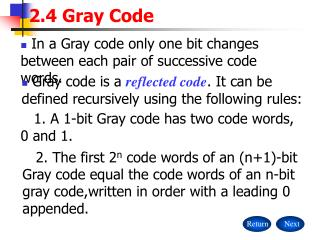DownloadDownload Presentation2.4 Gray Code

# 2.4 Gray Code

Download Presentation## 2.4 Gray Code

- - - - - - - - - - - - - - - - - - - - - - - - - - - E N D - - - - - - - - - - - - - - - - - - - - - - - - - - -
##### Presentation Transcript

1. 2.4 Gray Code Return Next • In a Gray code only one bit changes between each pair of successive code words. • Gray code is a reflected code. It can be defined recursively using the following rules: 1. A 1-bit Gray code has two code words, 0 and 1. 2. The first 2n code words of an (n+1)-bit Gray code equal the code words of an n-bit gray code,written in order with a leading 0 appended.

2. 2.4 Gray Code Return Back Next 3. The last 2n code words of an (n+1)-bit Gray code equal the code words of an n-bit gray code, but written in reverse order with a leading 1 appended. • Example: For a 2-bit Gray code, n=1. 2-bit Gray code 1-bit Gray code 00 01 00011110 01 11 10

3. 2.4 Gray Code Return Back • Example: For a 3-bit Gray code, n=2. 3-bit Gray code 00011110 0000 000001011010110111101100 2-bit Gray code 00011110 1111 10110100